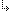- 4.45.2.25. -4. Standard Units4.45. Math - Extended Mathematics unit4.45.2. Math Unit Procedures and Functions4.45.2.25. DegToRad function

Targets: MS-DOS, OS/2, Win32

Math Unit

Returns the value of a degree measurement expressed in radians.

Declaration:
`function DegToRad(Degrees: Extended): Extended;`
Remarks:
Use DegToRad to convert angles expressed in degrees to the corresponding value in radians.
`  Radians = Degrees * PI / 180`